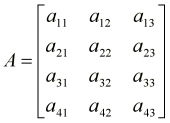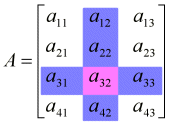2 min read

## #mastery28#TC1017

A matrix is, by definition, a rectangular array of numeric or algebraic quantities which are subject to mathematical operations. Matrices can be defined in terms of their dimensions (number of rows and columns). Let us take a look at a matrix with 4 rows and 3 columns (we denote it as a 4×3 matrix and call it A):Each individual item in a matrix is called a cell, and can be denoted by the particular row and column it resides in. For instance, in matrix A, element a32 can be found where the 3rd row and the 2nd column intersect.Matrices are used to represent complicated or time-consuming mathematical operations. A single matrix can hold an infinite number of calculations, which can then be applied to a number, vector, or another matrix. There are several operations that can be done on matrices, including addition, multiplication and inverse calculation; some of which will be discussed shortly. Operations done on one matrix can be transferred to another matrix simply by concatenating the two (by matrix multiplication). Matrices often find their use in 3 dimensional applications, were numerous identical operations are performed on thousands of vectors 30 or 40 times a second. Combining all these operations in one single matrix significantly improves the speed and functionality of a 3D rendering pipeline. Matrices are also used in financial processes (again, where a large number of data has to be processed in a similar fashion).